# 素数的那些事儿（三等奖）

1. 引子

2. 素数有多少？

N = p1p2…pn+1.

2,5,10,17, 26,…,n2 +1,…

3．素数是怎么分布的?

C1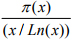≤C2, 这里C1, C2 是确定的常数.

π(x+y)≤π(x)+π(y) , 这里π(x)同前定义.

4. 如何构造素数？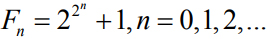Mp -1, p=2,3,5,7 …

n2 – n + p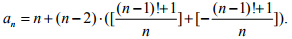5. 素数和方程

(1) 我们考虑不定方程

x(x-1)…(x- (p-1)) = py.

(2) 费马小定理断言: 不定方程

Xn + Yn = Zn, n≥3.

(3) 假设n 是一个整数, 并且不为p 整除. 我们要求不定方程

nx – py =1

(4)(平方剩余) 求解以下方程的整数解是初等数论的核心问题之一：

x2 – py = q

x2 – 3y = 2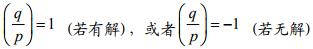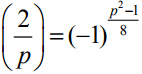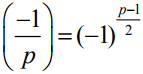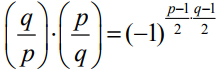x2 – py = q，z2 – qw = p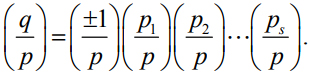(5)两平方和问题: 一个素数p 什么时候可以写成两个平方数之和? 比如

5 = 12 + 22, 13 = 22 + 32 , …

x2 + y2 = p.

pz2=(xz)2+(yz)2=(xz)2+(yz-1+1)2 = (xz)2+1+ 2(yz-1)+(yz-1)2.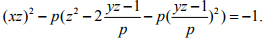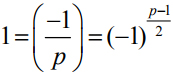（1）a,b,c 是整数, 并且两两之间互素（两个数互素，是指不存在大于1 的整数能同时整除它们）

（2）a+b=c

（3）c > d1+ε , 这里d 是abc 中所有互不相同的素因子的乘积.

6.素数和代数

a + b√-1,这里a,b 都取整数.所有这些复数构成的集合记为Z[√-1]. 我们可以像整数一样定义高斯整数的加减乘运算, 甚至我们还可以定义两个高斯整数的带余数除法等. 完全类似地, 我们自然也可以定义Z[√-1]中的“素数”概念.

p = a2 + b2.

p = (a + b√-1)(a – b√-1).

(1) P =1+ √-1;

(2) P = a + b√-1, 使得p = a2 + b2是整数集合里被4 除余数为1 的素数;

(3) P 就是整数集合里被4 除余数为3 的素数.

Q[√ m] = {a + b√m | a,b∈Q},

Q[√m]中的“整数”是什么样的呢? 答案与我们想象的稍微不同:

a + b√m, 这里 a,b 是整数.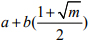, 这里 a,b 是整数.

21 = 3·7 = (1+ 2√-5)· (1- 2√-5).

Xn +Yn = Zn

7.素数和函数

(1)对任何介于0 和1 之间的实数x, 都有无限求和公式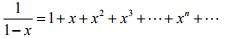(2)将下式左边展开并利用算术基本定理得到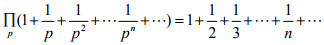(3) 结合(1)(2), 则有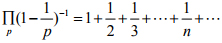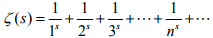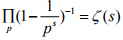ζ(s) = 0.

ζ(s)的非平凡零点一定可以写成形如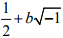的复数(b 是实数).换言之，所有的非平凡零点都落在直线Re(s)=1/2 上， 这里Re 表示取复数的实部.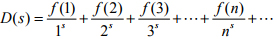8. 小结

～～～欢迎转发～～～

！！！转载请联系我们获取授权！！！